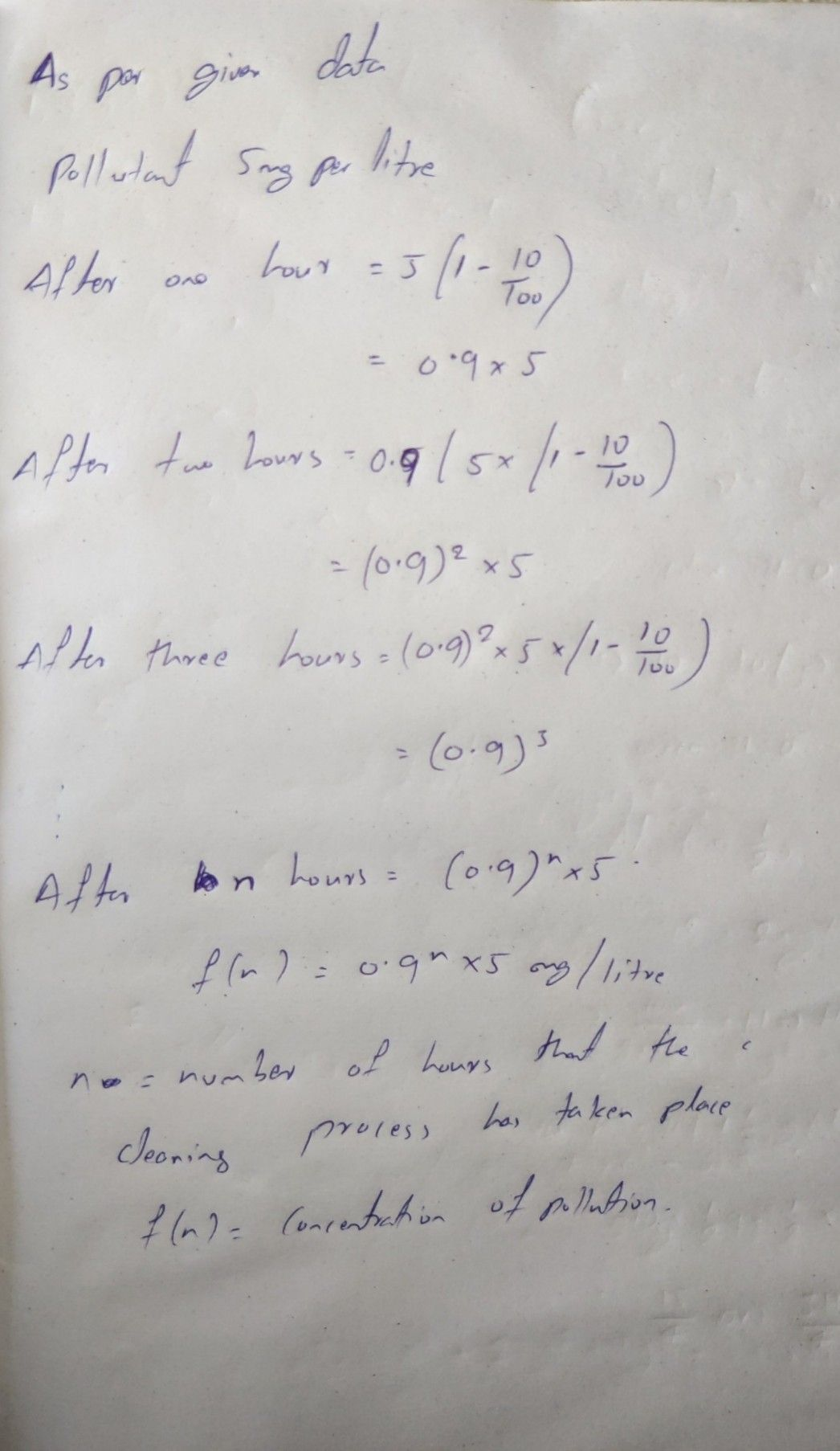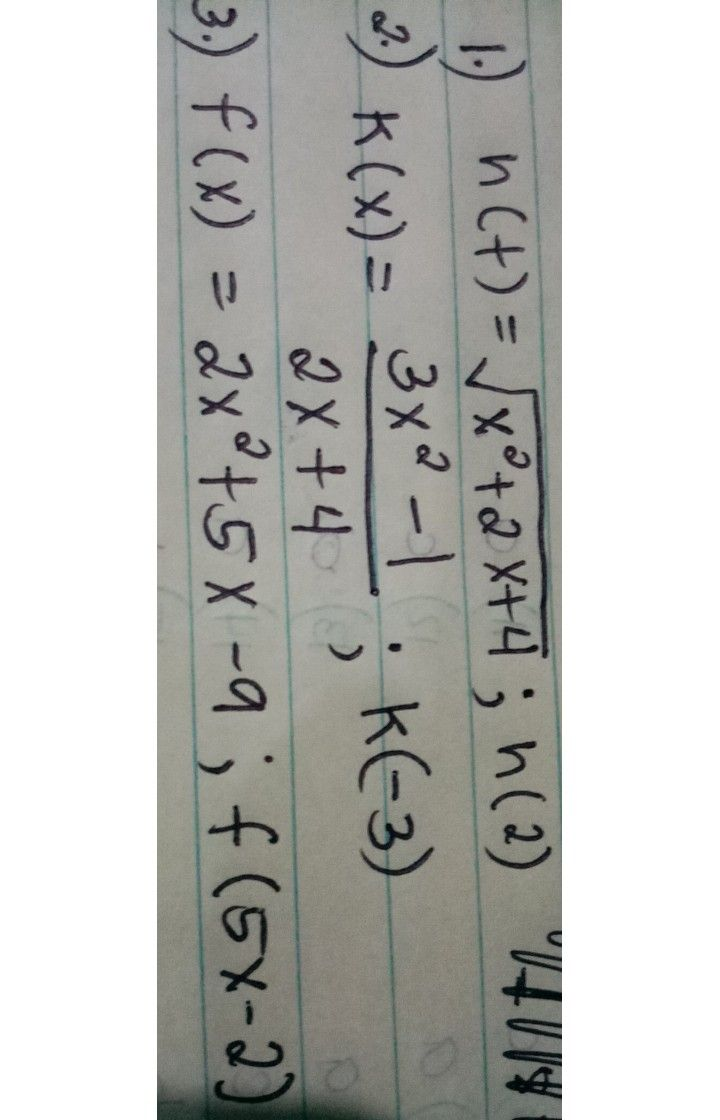Symbol
ProblemRead and $aaaly2e$ each situation carefully and apply $y$ your learnings on $xepxe8eaimg$ $\pi ea1-H\pi e$ situations involving functions including piecewise. $1.$ Contaminated water is subjected to a cleaning process. The concentration of the pollutants is initially $5$ mg $2$ per liter of water. If the cleaning $B$ process $C$ can reduce the pollutant by $10%$ each hour, $leGme$ $9$ function that $Q$ can represent the concentration of pollutants in the water in terms of the number of hours that the cleaning $Pxoo6ss$ has taken place.
10th-13th grade
Geometry
Search count: 106
SolutionQanda teacher - AshrithaInitial concentration of untreated or polluted water water =  5 mg per litre Pollutants reduce by 10% every hour Pollutants remaining after an hour would be = (100-10)/100 x 5 Pollutants remaining after an hour would be = (0.9) x 5 = 4.5 Pollutants remaining after two hours would be = 0.9 x (0.9) x 5 Pollutants remaining after nth hour of treatment would be = 0.9^n x 5Student